# Finding Derivatives of Sums, Products, Differences & Quotients

Instructor: Laura Pennington

Laura received her Master's degree in Pure Mathematics from Michigan State University, and her Bachelor's degree in Mathematics from Grand Valley State University. She has 20 years of experience teaching collegiate mathematics at various institutions.

This lesson will go over how to find the derivative of a sum, difference, product, and quotient. We will look at the different formulas involved in these derivatives and use those formulas to calculate some derivatives.

## Working with Derivatives

Suppose you have a job for a company that builds cars. Your boss tells you they are working on a car part, and a certain aspect of it can be represented by one of the following four functions.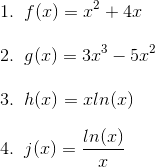You're told that you need to be able to find the slope of each of these functions for any given value of x. You remember that the derivative of a function represents the slope of that function at any given point. Great! All you have to do is find the derivative of each of these functions and your boss will be a happy camper.

You look at each of the functions and realize that the first function is a sum of functions, the second function is a difference of functions, the third function is a product of functions, and the fourth function is a quotient of functions. Thus, we want to look at how to find the derivative of a sum, difference, product and quotient. Let's get started!

## Derivative of a Sum

When calculating the derivative of a sum, we simply take the sum of the derivatives. This is illustrated in the following formula.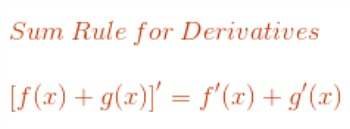The first function that your boss wants you to work with is the sum of two functions. Therefore, to find the derivative of this function, we just take the sum of the derivatives. To do this, we need to recognize that the derivative of x 2 is 2x, and the derivative of 4x is 4. Now we just plug into our formula and we've got our derivative.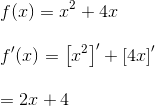We see that the derivative of the first function is f ' (x) = 2x + 4.

## Derivative of a Difference

The second function, g, is a difference of functions. When we want to find the derivative of a difference, we simply find the difference of the derivatives. This is similar to the sum rule, and is shown in the following formula.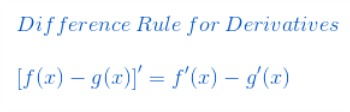To use our formula for our example, we just need to know that the derivative of 3x3 is 9x 2, and the derivative of 5x 2 is 10x. Once again, we just plug into our formula.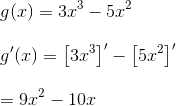Using our formula, we've found that the derivative of the second function is g' (x) = 9x 2 - 10x.

## Derivative of a Product

Now things get a little trickier. The third function is a product of the functions x and ln(x). When we want to find the derivative of a product, we use the product rule for derivatives.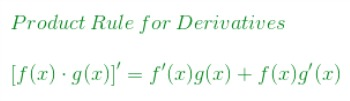Thus, to find the derivative of our function h in our example, we need to know that the derivative of x is 1, and the derivative of ln(x) is 1/x, and then we just need to use our formula.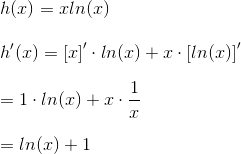We see that the derivative of the third function is h' (x) = ln(x) + 1.

To unlock this lesson you must be a Study.com Member.
Create your account

### Register to view this lesson

Are you a student or a teacher?

### Unlock Your Education

#### See for yourself why 30 million people use Study.com

##### Become a Study.com member and start learning now.
Back
What teachers are saying about Study.com
Create an account to start this course today
Used by over 30 million students worldwide